## Thursday, 7 August 2014

### chapter-16 Worked Out Examples

 Example: 13
 In an objective type examination, each question has four options of which only one is correct. A student taking the examination either guesses or copies or knows the answer to any question. The probability that he guesses is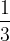$\dfrac{1}{3}$ and that the he copies is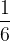$\dfrac{1}{6}$. The probability that he answers correctly if he copies is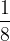$\dfrac{1}{8}$. If an answer is given to be correct, find the probability that the student knew the answer.
 Solution: 13
We see that the student can make three moves, with associated probabilities as: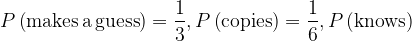$P\left( {{\rm{makes \,a \,guess}}} \right) = \dfrac{1}{3},P\left( {{\rm{copies}}} \right) = \dfrac{1}{6},P\left( {{\rm{knows}}} \right)$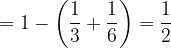$= 1 - \left( {\dfrac{1}{3} + \dfrac{1}{6}} \right) = \dfrac{1}{2}$
Now, if he copies, we know that the probability of his answer being correct is$\dfrac{1}{8}$. Also, if he guesses, he’ll select any of the four options with equal likelihood, which means that the probability of his answer being correct is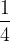$\dfrac{1}{4}$.
Thus, we have the following probability tree:We see that we have three paths leading to the correct answer, and we want to find the probability of the bottomost path, that is the probability that the student knew the answer given that he answered it correctly. This probability is now simply: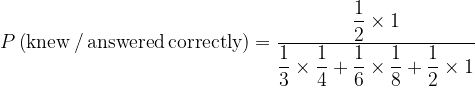$P\left( {{\rm{knew \,/ \,answered\, correctly}}} \right) = \dfrac{{\dfrac{1}{2} \times 1}}{{\dfrac{1}{3} \times \dfrac{1}{4} + \dfrac{1}{6} \times \dfrac{1}{8} + \dfrac{1}{2} \times 1}}$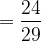$= \dfrac{{24}}{{29}}$
Can you appreciate why this value is so high (greater than$80\%$)?
 Example: 14
 A speaks truth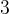$3$ times out of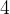$4$ while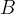$B$ does so$7$ times out of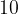$10$. A ball is drawn at random from a bag containing one black ball and five other balls of different colors. Both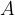$A$ and$B$ report that a black ball has been drawn from the bag. Find the probability of their assertion being true.
 Solution: 14
To slightly rephrase the problem, we’ve to find the probability of the ball actually being black given that both$A$ and$B$ report it to be black.
The probability tree can easily be drawn (partially shown here, only the paths relevant to us have been completed):We want to find the probability of the upper path which is: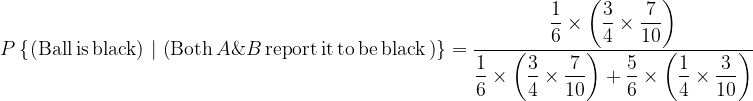$P\left\{ {\left. {\left( {{\rm{Ball\, is\, black}}} \right)\,\,} \right|\,\left( {{\rm{Both}}\,A\& B\,{\rm{report\, it\, to\, be\, black\,}}} \right)} \right\} = \dfrac{{\dfrac{1}{6} \times \left( {\dfrac{3}{4} \times \dfrac{7}{{10}}} \right)}}{{\dfrac{1}{6} \times \left( {\dfrac{3}{4} \times \dfrac{7}{{10}}} \right) + \dfrac{5}{6} \times \left( {\dfrac{1}{4} \times \dfrac{3}{{10}}} \right)}}$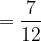$= \dfrac{7}{{12}}$
Let us now rewrite the solution in standard terminology. Let us define the following events:$B$ : Black ball is drawn from the bag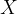$X$ : Both$A$ and$B$ report black
Thus, observe that we need to find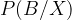$P(B/X)$ which is by the Baye’s theorem (in fact compare this relation with the tree diagram in Fig-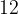$12$ and see how the two correspond)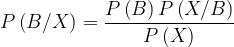$P\left( {B/X} \right) = \dfrac{{P\left( B \right)P\left( {X/B} \right)}}{{P\left( X \right)}}$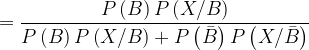$= \dfrac{{P\left( B \right)P\left( {X/B} \right)}}{{P\left( B \right)P\left( {X/B} \right) + P\left( {\bar B} \right)P\left( {X/\bar B} \right)}}$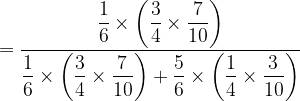$= \dfrac{{\dfrac{1}{6} \times \left( {\dfrac{3}{4} \times \dfrac{7}{{10}}} \right)}}{{\dfrac{1}{6} \times \left( {\dfrac{3}{4} \times \dfrac{7}{{10}}} \right) + \dfrac{5}{6} \times \left( {\dfrac{1}{4} \times \dfrac{3}{{10}}} \right)}}$$= \dfrac{7}{{12}}$